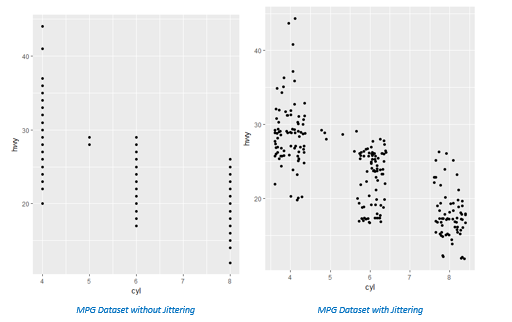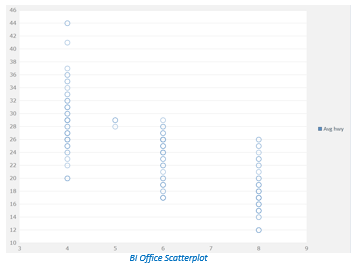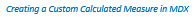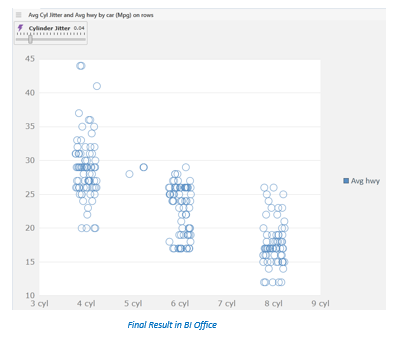1

# Creating Jittered Scatterplots in BI Office 6

by Tim Andrews , Power User of BI Office

When tasked with visualizing a relationship between a continuous and more discrete variable, a jittered scatterplot can be a necessary evil.  Used regularly by many analysts, the jittering effect is built into the ggplot2 library in R.  By plotting the MPG dataset in R (cylinder count by highway MPG), we can quickly see that data points are lying directly on top of each other, masking information about the number of observations and highway MPG clusters.  The jittering effect provides more clarity.A similar effect can be accomplished in BI Office 6 by using custom calculated measures and MDX.   Using the same MPG dataset, we are able to render a scattlerplot in BI Office.  This scatterplot suffers from the same design challenges as the first R plot – much of the interesting information is masked on the x axis when we use an integer value such as the number of cylinders.  We can clearly see that we have many observations for 4, 6, and 8-cylinder engines, along with probably fewer 5-cylinder engines.  We don’t have a good read on exactly how many cars we are measuring, or if there are clusters against certain MPG levels.## The Solution: A New Calculated Measure

The solution requires us to create a new calculated measure for cylinder count – and introduce some random noise to the exact value in order to horizontally spread our data points.  My implementation also contains an optional variable to allow the analyst or end-user to alter the severity of the jitter.

--Random number between our MIN and MAX jitter, AS a percentage

(((  [Variable].[!##Cylinder Jitter##!] - (  [Variable].[!##Cylinder Jitter##!] * -1)) * RND(RANK([Mpg].[car].CURRENTMEMBER, [Mpg].[car].ALLMEMBERS)) + (  [Variable].[!##Cylinder Jitter##!] * -1))

*

--Multiply the random number by the average of all cylinders to capture the offset

AVG([Mpg].[car].[All].CHILDREN *  [Measures].[Avg cyl] ))

+

--Add the offset to our cylinder VALUE

[Measures].[Avg cyl]To explain what’s happening in the calculation, let’s start at the beginning.

First, a random number between an upper and lower limit is generated.  The standard VBA/MDX Rnd() function is utilized.    In my example, I am using a variable and the negative of the variable to determine the upper and lower limits for the jitter.  My default for the variable is .05, as I am expressing the jitter as a percentage.  The limits could be hard-coded if desired.  Since the Rnd function returns the same number for all rows in a cellset, a unique number for each member is generated with the RANK statement and passed in as a seed value.  Thanks to Tomislav Piasevoli's BI Blog for the idea for generating unique random numbers per row:  http://tomislav.piasevoli.com/category/mdx/.

Next, the random number is multiplied by the average cylinder count for all cars in the dataset, providing a random offset.  This is an important step as it ensures a uniform jitter pattern across all cylinder counts.  If the percentage was used individually against 4 and 8-cylinder engines, the spread would appear non-uniform.

Lastly, the result of the first two steps is added to the actual cylinder value to incorporate the offset.

As an added bonus, I altered the format for the new calculation to display the name of the cylinder metric along the x-axis (the format expression being “0 cyl”).

Here is the final result.  Note the jitter variable control above the plot.  Using a random jitter of +/- 4%, the scatterplot now provides more useful information, and we can quickly see clusters emerge, such as the 25 to 30-MPG range for 4-cylinder engines.Thank you, Tim, for this great article! If you would like to be featured on the community, message me for more information!